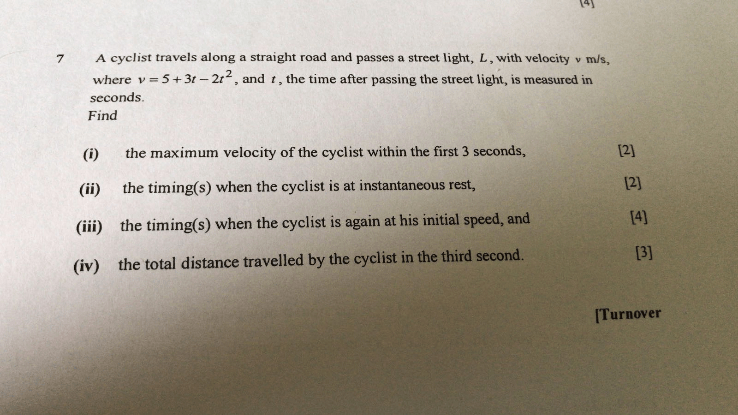# QuestionHi! Can anyone help me with part iii and iv of the qns? Thanks so much!

This is a differentiation question.

a)

v  = 5 + 3t – 2t^2

dv/dt = 3 – 4t = 0

t = 3/4

v = 5 + 3 (3/4) – 2 (3/4)^2 = 16.125

b)

t = 3/4

c)

Integrate v = 0

d = 5t + 3/2 t^2 – 2/3 t^3 = 0

t(5 + 3/2 t – 2/3 t^2) = 0

t = 0, 4.089, -1.836 (rej)

d)

Integrate v (0  3) – integrate v (3   4.089)

= …

0 Replies 1 Like